Here is our velocity equation: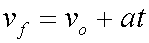There are other popular ways to write the symbols in this equation.

More that one symbol exists for the original velocity. All three of these symbols are the same idea:

 vo Original velocity vi Initial velocity v1 First velocity

And there is more that one symbol for the final velocity. Both of these symbols mean the same thing:

 vf Final velocity v2 Second velocity

Basically, these velocity symbols fall into two families.

These go together in one family:

vo, vi, and vf

These go together in another family:

v1 and v2

So, using the first family of velocity symbols, note that these two equations mean the same: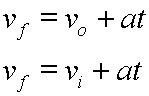Using the second set of symbols, again, we say the identical idea with this notation: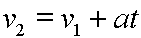When you write the equation, you would never mix a symbol from one family with a symbol from another. So, you would not write something like this: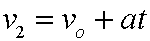A very good way to think about our velocity equation is that it will give you the velocity of the object, (final velocity), at any given time. You would need to know the original velocity, of course. That would be a known fixed quantity, or constant.

That is, our velocity equation can be thought of as a function. It would be velocity as a function of time. In function notation, the equation would look like this: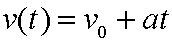Custom Search